Home > FFT 2020

# The Norbert Wiener Center Online Seminar on Harmonic Analysis and Applications

FFT 2020 Home

### Practical Matters

Getting to the FFT

Organizing Committee and Contacts

Previous FFTs

When:

Mondays at 11 a.m. PST / 2 p.m. EST / 8 p.m. CET

Where:

Recorded talks will be available on Youtube channel.

# Upcoming Talks:

 November 30th, 2020 Carlos Cabrelli (University of Buenos Aires) Frames by Operator Orbits I will review some results on the question of when the orbits {Tj g: j ∈ J, g ∈ G}, of a bounded operator T acting on a Hilbert space H where G is a subset of H forming a frame of H. I will also comment on recent advances. This is motivated by the Dynamical Sampling problem that consists of recovering a time-evolving signal from its space-time samples. December 7th, 2020 Palle Jorgensen (University of Iowa) December 14th, 2020 Gil Strang (MIT) December 21st, 2020 Vivek Goyal (Boston University) January 4th, 2021 Qiyu Sun (University of Central Florida) January 11th, 2021 Victor Wickerhauser (Washington University in St. Louis) January 25th, 2021 Andrea Bertozzi (UCLA) February 1st, 2021 Michael Lacey (Georgia Tech) February 8th, 2021 Alfred Hero (University of Michigan) February 15th, 2021 Akram Aldroubi (Vanderbilt University) February 22nd, 2021 Pete Casazza (University of Missouri) March 1st, 2021 Marcin Bownik (University of Oregon) March 8th, 2021 Rodolfo Torres (University of California, Riverside)

# Previous Talks:

 November 23rd, 2020 Tomaso Poggio (MIT) Deep Puzzles: Towards a Theoretical Understanding of Deep Learning Very recently, square loss has been observed to perform well in classification tasks with deep networks. However, a theoretical justification is lacking, unlike the cross-entropy case for which an asymptotic analysis is available. Here we discuss several observations on the dynamics of gradient flow under the square loss in ReLU networks. We show how convergence to a local minimum norm solution is expected when normalization techniques such as Batch Normalization (BN) or Weight Normalization (WN) are used, in a way which is similar to the behavior of linear degenerate networks under gradient descent (GD), though the reason for zero-initial conditions is different. The main property of the minimizer that bounds its expected error is its norm: we prove that among all the interpolating solutions, the ones associated with smaller Frobenius norms of the weight matrices have better margin and better bounds on the expected classification error. The theory yields several predictions, including aspects of Donoho's Neural Collapse and the bias induced by BN on the weight matrices towards orthogonality. November 16th, 2020 Jean Pierre Gabardo (McMaster University) Factorization of positive definite functions through convolution and the Turan problem An open neighborhood U of 0 in Euclidean space is called symmetric if -U=U. Let PD(U) be the class of continuous positive definite functions supported on U and taking the value 1 at the origin. The Turan problem for U consists in computing the Turan constant of U, which is the supremum of the integrals of the functions in PD(U). Clearly, this problem can also be stated on any locally compact abelian group. In this talk, we will introduce the notion of "dual" Turan problem. In the case of a finite abelian group G, the Turan problem for a symmetric set S consists thus in maximizing the integral (which is just a finite sum) over G of the positive definite functions taking the value 1 at 0 and supported on S, while its dual is just the Turan problem for the set consisting of the complement of S together with the origin. We will show a surprising relationship between the maximizers of the Turan problem and those of the dual problem. In particular, their convolution product must be identically 1 on G. We then extend those results to Euclidean space by first finding an appropriate notion of dual Turan problem in this context. We will also point out an interesting connection between the Turan problem and frame theory by characterizing so-called Turan domains as domains admitting Parseval frames of (weighted) exponentials of a special kind. November 9th, 2020 Jill Pipher (Brown University) Boundary value problems for elliptic complex coefficient systems: the p-ellipticity condition Formulating and solving boundary value problems for divergence form real elliptic equations has been an active and productive area of research ever since the foundational work of De Giorgi - Nash - Moser established Holder continuity of solutions when the coefficients are merely bounded and measurable. The solutions to such real-valued equations share some important properties with harmonic functions: maximum principles, Harnack principles, and estimates up to the boundary that enable one to solve Dirichlet problems in the classical sense of nontangential convergence. Solutions to complex elliptic equations and elliptic systems do not necessarily share these good properties of continuity or maximum principles. In joint work with M. Dindos, we introduce in 2017 a structural condition (p-ellipticity) on divergence form elliptic equations with complex valued matrices which was inspired by a condition related to Lp contractivity due to Cialdea and Maz'ya. The p-ellipticity condition that generalizes Cialdea-Maz'ya was also simultaneously discovered by Carbonaro-Dragicevic, who used it to prove a bilinear embedding result. Subsequently, Feneuil - Mayboroda - Zhao have used p-ellipticity to study well-posedness of a degenerate elliptic operator associated with domains with lower-dimensional boundary. In this seminar, we discuss p-ellipticity for complex divergence form equations, and then describe recent work, joint with J. Li and M. Dindos, extending this condition to elliptic systems. In particular, we can give applications to solvability of Dirichlet problems for the Lame systems. November 2nd, 2020 Ursula Molter (University of Buenos Aires) Riesz Bases of Exponentials and the Bohr Topology In this talk we address the question of what domains Ω of Rd with finite measure, admit a Riesz basis of exponentials, that is, the existence of a discrete set B ⊂ Rd such that the exponentials E(B) = {e2piß·ω : ß ∈ B} form a Riesz basis of L2(Ω). Using the Bohr compactification of the integers, we show a necessary and sufficient condition to ensure that a multi-tile Ω subset of Rd of positive measure (but not necessarily bounded) admits a structured Riesz basis of exponentials for L2(Ω). Here a set Ω ⊂ Rd is a k-multi-tile for Zd if Σλ ∈ Zd ΧΩ(ω - λ) = k a.e. ω ∈ Rd. October 26th, 2020 Virginia Naibo (Kansas State University) Fractional Leibniz Rules: A Guided Tour The usual Leibniz rules express the derivative of a product of functions in terms of the derivatives of each of the factors. In an analogous sense, fractional Leibniz rules involve the concept of fractional derivative and provide estimates of the size and smoothness of a product of functions in terms of the size and smoothness of each of the factors. These bilinear estimates stem from the study of partial differential equations such as Euler, Navier Stokes and Korteweg-de Vries. In this talk, I will present fractional Leibniz rules associated to bilinear pseudodifferential operators with homogeneous symbols, including Coifman-Meyer multipliers, and with symbols in the bilinear Hörmander classes. Through different approaches, the estimates will be discussed in the settings of weighted Lebesgue, Triebel-Lizorkin and Besov spaces. October 19th, 2020 Ronald Coifman (Yale) Phase Unwinding Analysis: Nonlinear Fourier Transforms and Complex Dynmaics Our goal here is to introduce recent developments of analysis of highly oscillatory functions. In particular we will sketch methods extending conventional Fourier analysis, exploiting both phase and amplitudes of holomorphic functions. The miracles of nonlinear complex holomorphic analysis, such as factorization and composition of functions lead to new versions of holomorphic orthonormal bases , relating them to multiscale dynamical systems, obtained by composing Blaschke factors. We also, remark, that the phase of a Blaschke product is a one-layer neural net with (arctan as an activation sigmoid) and that the composition is a "Deep Neural Net" whose depth is the number of compositions, our results provide a wealth of related libraries of orthogonal bases . We will also indicate a number of applications in medical signal processing , as well in precision Doppler. Each droplet in the phase image below represent a unit of a two layers deep net and gives rise to an orthonormal basis the Hardy space October 12th, 2020 Alex Iosevich (University of Rochester) Finite Point Configurations and Applications to Frame Theory We are going to discuss some recent developments in the study of finite point configuration in sets of a given Hausdorff dimension. We shall also survey some applications of the finite point configuration machinery to the problems of existence and non-existence of exponential/Gabor bases and frames.

Organizing Committee:
Wojtek Czaja
Jacob Bedrossian
John Benedetto
Vince Lyzinski
Thomas Goldstein
Ray Schram

In cooperation with

Now in Print!
Excursions in Harmonic Analysis:
The Fall Fourier Talks at the Norbert Wiener Center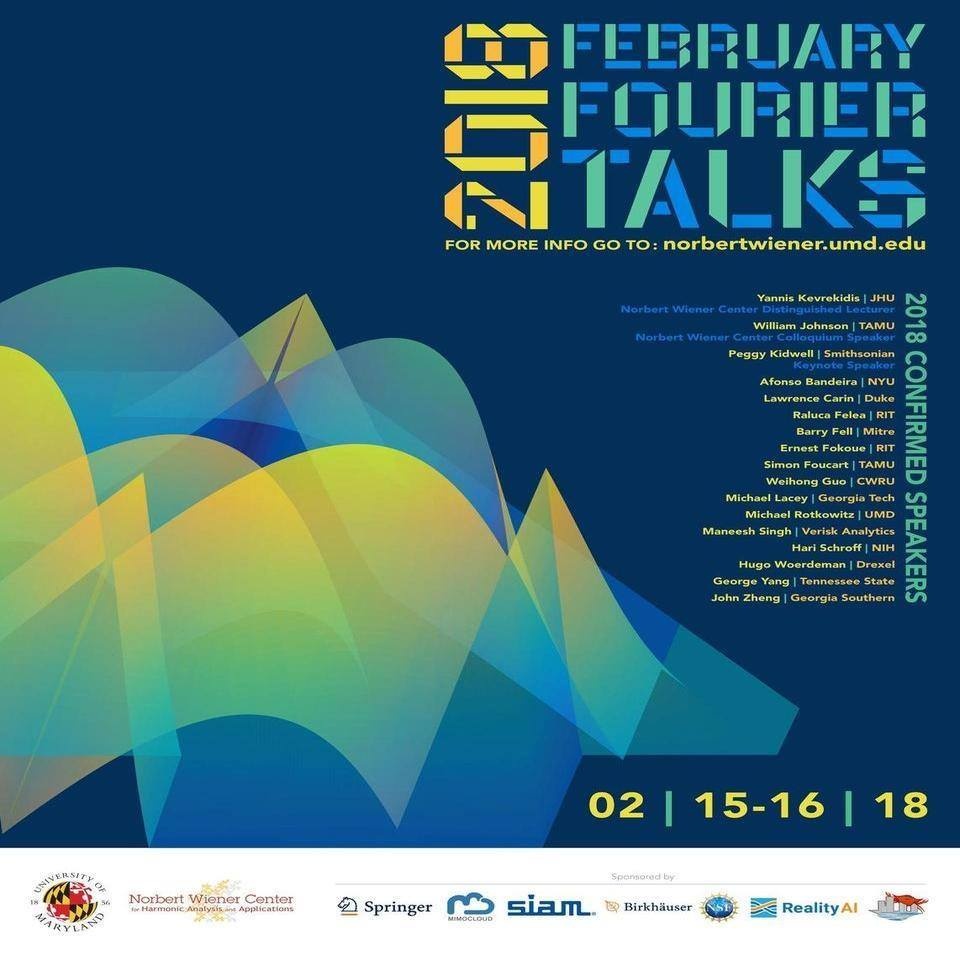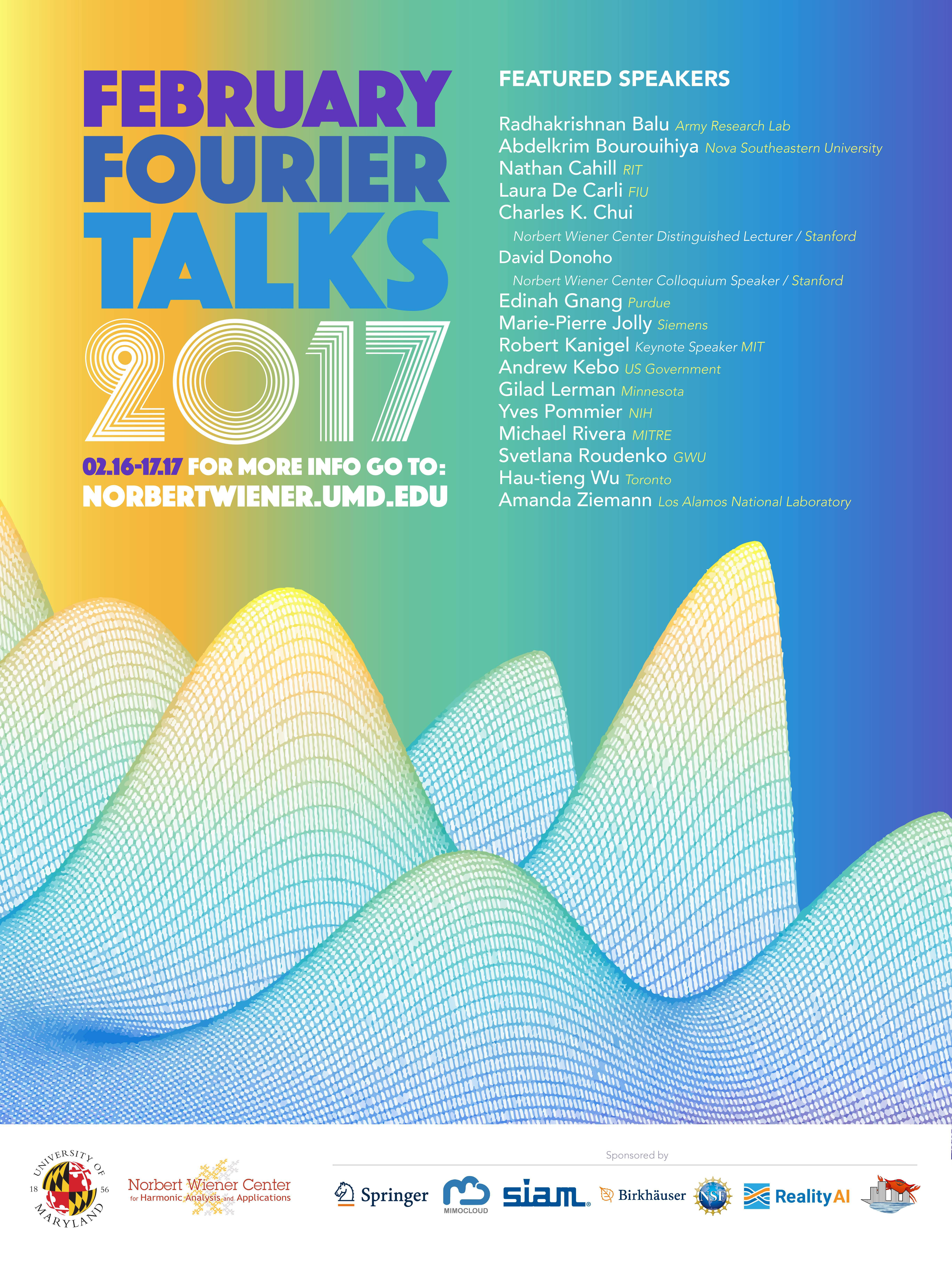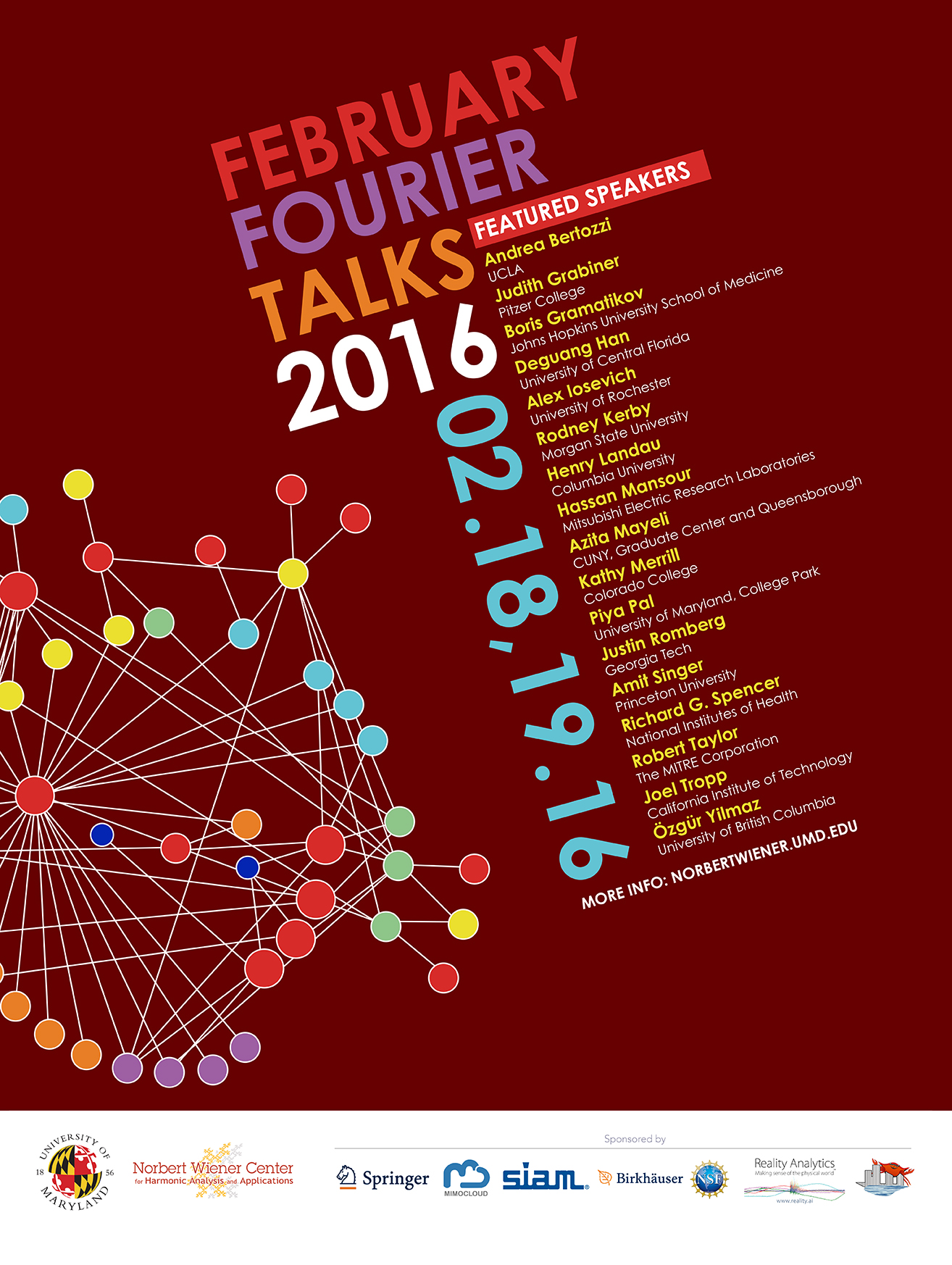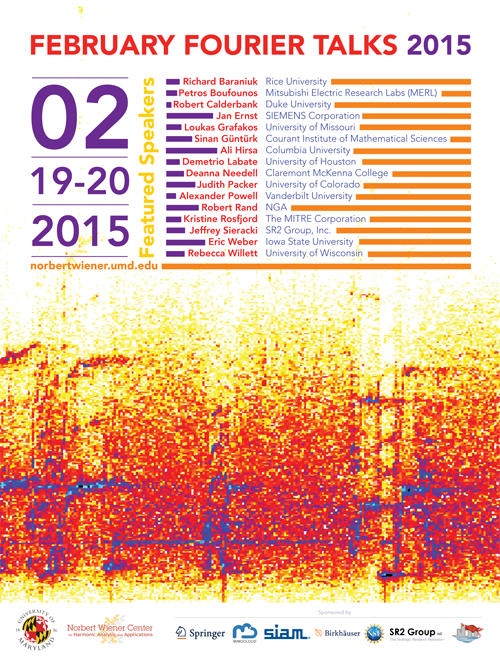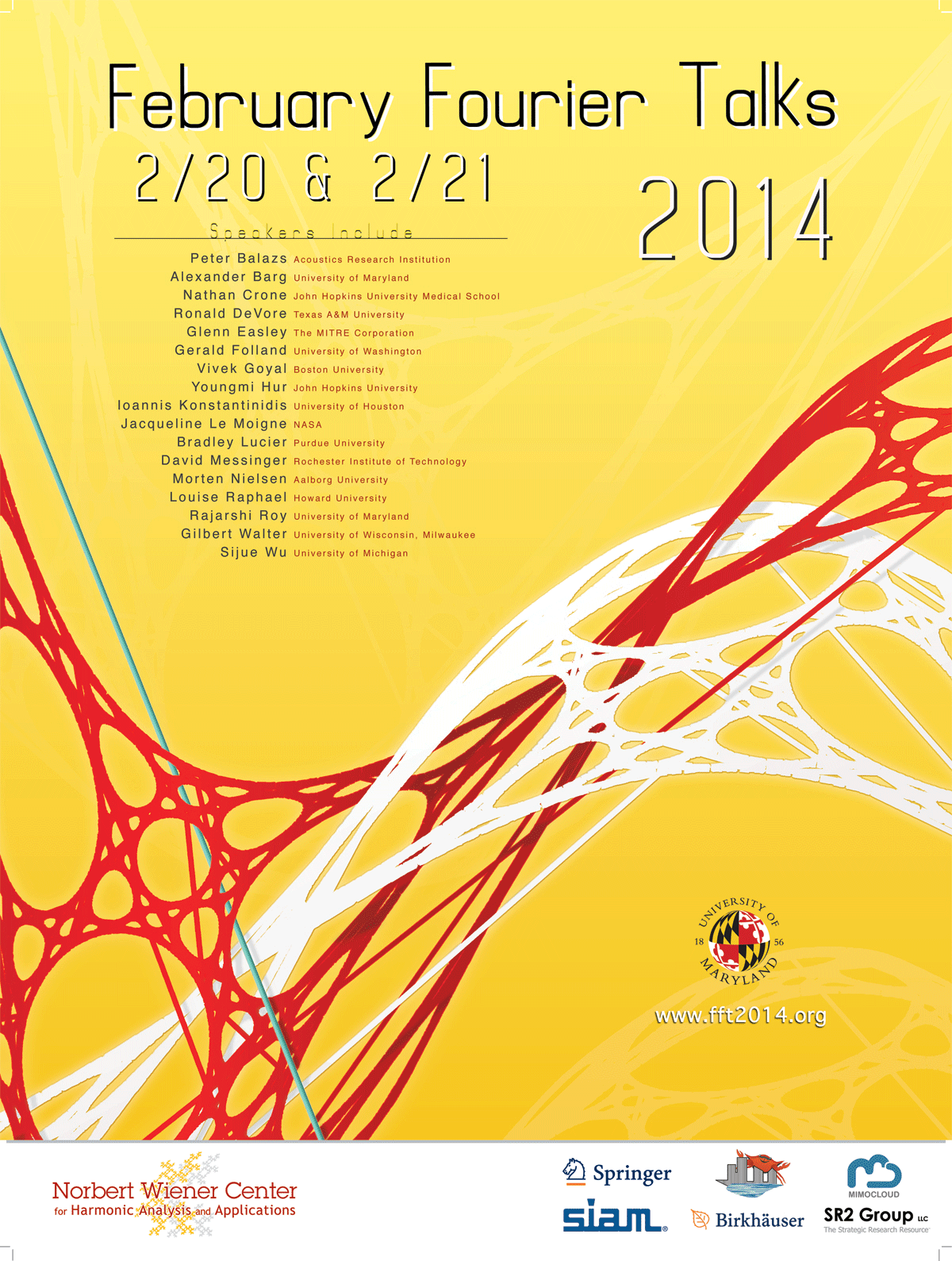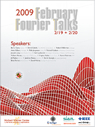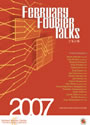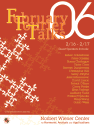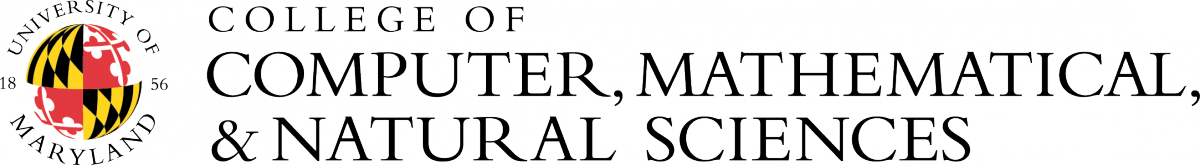### Stay ConnectedNorbert Wiener Center
Department of Mathematics
University of Maryland
College Park, MD 20742
Phone: (301) 405-5158
The Norbert Wiener Center is part of the College of Computer, Mathematical, and Natural Sciences.